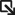• Solutions Solutions
• Products Products
• Services Services
• Support SupportSupport Portal ›
• Resources Resources
##### Networking Made Simple, Easy, and Affordable - Q&A with the Senior Director of Extreme Capital Solutions
All stories ›
• Partners Partners

# Ratio Expression

A CLEAR-Flow ratio expression compares the ratio of two counter values with the threshold value.

The following is the syntax for a CLEAR-Flow ratio expression:

```ratio <counterNameA> <counterNameB> REL_OPER <countThreshold> ;
min-value <min-value> ;
hysteresis <hysteresis> ;
```

The values of countThreshold and hysteresis can be specified as floating point numbers, and the ratio is computed as a floating point number. The ratio statement specifies how to compare the ratio of two counters with its threshold. To compute the ratio, the value of counterNameA is divided by the value of counterNameB. That ratio is then compared with the countThreshold. The REL_OPER is selected from the relational operators for greater than, greater than or equal to, less than, or less than or equal to (>, >=, <, <=).

The min-value statement is optional, and sets a minimum value for the counters. If either counter is less than the minimum value, the expression evaluates to false. If not specified, the minimum value is 1.

The hysteresis hysteresis statement is optional and sets a hysteresis value for the threshold. After the ratio statement is true, the value of the threshold is adjusted so that a change smaller than the hysteresis value will not cause the statement to become false. For statements using the REL_OPER > or >=, the hysteresis value is subtracted from the threshold; for < or <=, the hysteresis value is added to the threshold.

For example, the following ratio expression:

```ratio counter1 counter2 >= 5 ;
min-value 100;
hysteresis 1 ;
```

is true only after the ratio of the counters reaches at least 5 and the counter values are at least 100. At the time it became true, the hysteresis value would be subtracted from the threshold (setting the threshold to 4). With the threshold now at 4, the condition would stay true until the ratio of the counters became less than 4.

If the statement becomes false, the threshold is reset to its original value. You can use the hysteresis value to prevent the rule from vacillating between the true and false states if the ratio between the counter values is near the threshold. If the hysteresis value is greater than the threshold value, the hysteresis value will be set to 0.

The following table is an example of evaluating the CLEAR-Flow ratio expression above multiple times. Notice that the rule is not triggered at the first evaluation because both counters have not yet reached the min-value of 100. The rule first triggers at evaluation 3, when ratio of the two counters exceeds 5. After the rule is triggered, it remains triggered until the ratio value is less than 4 (the original threshold minus the hysteresis), at evaluation 5. At evaluation 7, the rule is again triggered when the ratio reaches 5. The rule will remain triggered until the ratio drops below 4.Ratio Expression Evaluation Example

Evaluation counter1 value counter2 value Ratio Rule Triggered?
1 427 70 6 No
2 941 235 4 No
3 2475 412 6 Yes
4 2308 570 4 Yes
5 2313 771 3 No
6 3597 899 4 No
7 5340 1065 5 Yes

See Ratio Expression Example for a full example of an ACL and a CLEAR-Flow rule using a ratio expression.